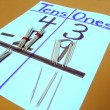### Blog Archives

•### Math - Popsicle Stick Regrouping Fun

Objectives: Learning and practising regrouping Print examples of regrouping in student math journals Learn term names:  minuend take away subtrahend equals difference/answer (for subtraction) and addend plus addend equals sum/answer (for addition) Materials for each group … View Full Lesson →

• ### Math - Put the Order in Ordinal Numbers

Prerequisite: Practise reading numbers from the Hundreds Chart, especially the first 31 numbers. Objective: Learning to put ordinal numbers in order Learning:   Ordinal numbers: numbers that tell their position in a series.  They end in ”  st,   … View Full Lesson →